## 22nd Century military units (Space Force)

Moderator: NoXion

NoXion
Posts: 556
Joined: Tue Jun 24, 2008 6:51 pm
Location: Nova Mundi

### 22nd Century military units (Space Force)

SPACE FORCE UNITS

Cost of upkeep is = total military Cost in energy divided by four. The following are stats for one unit each of:

Size Class A: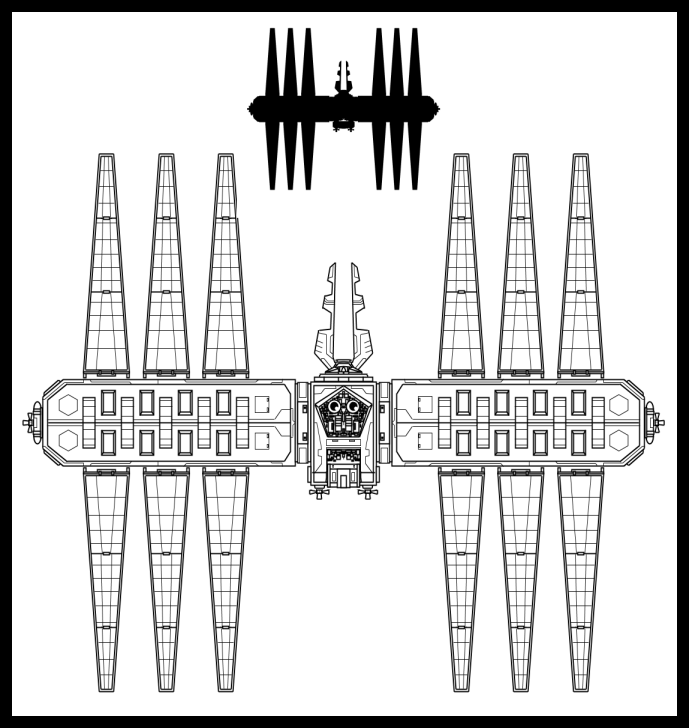Ground Targeting Satellite
At: 10
Df: 5
Mv: Orbit
Cost: 4e+4r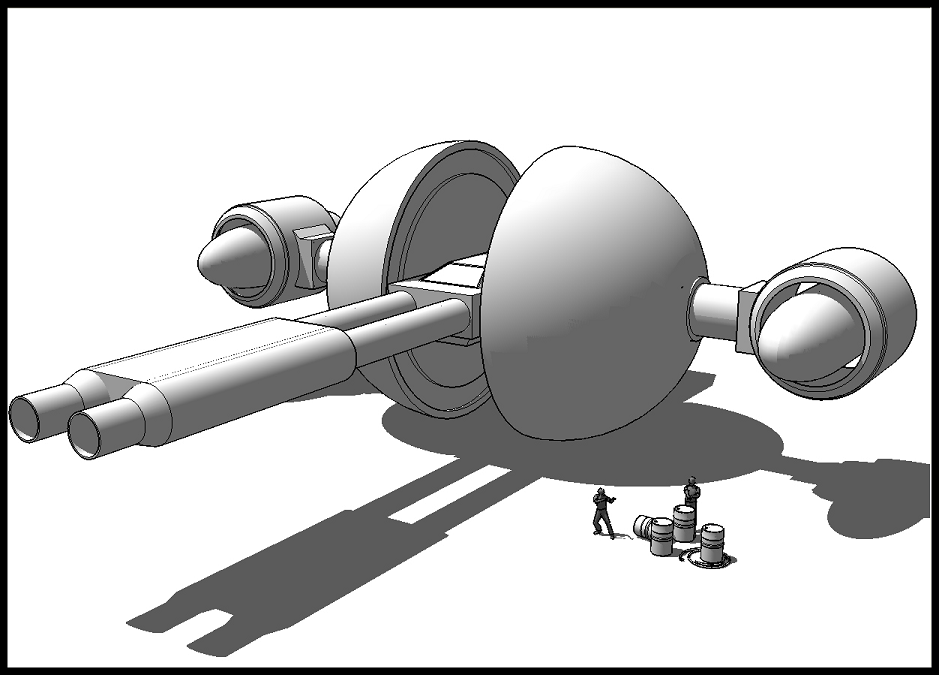Anti-Orbital Drone
At: 20
Df: 10
Mv: Orbit
Cost: 4e+4r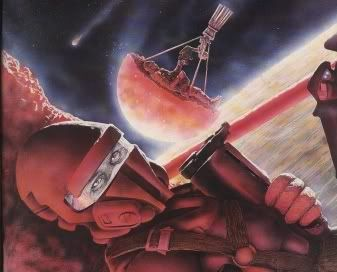Espatiers
At: 10
Df: 8
Mv: Orbital assault/infiltration, foot
Cost: 4e+4r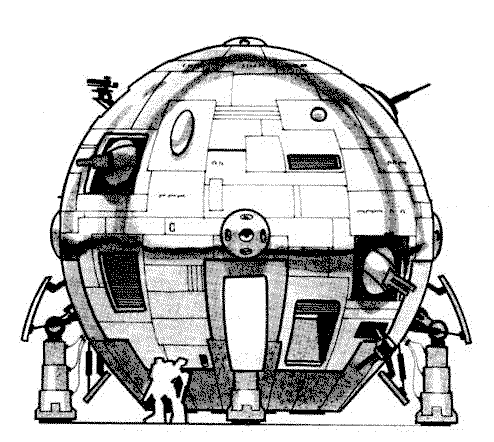Dropship
At: 10
Df: 20
Mv: Surface lander, surface to orbit
Cost: 8e+8r
Note: Can carry up to 2 roughly tank-sized units and 6 infantry units.Space Fighter
At: 15
Df: 10
Mv: Trans-orbital plasma engines (Cislunar space, Jovian moons etc)
Cost: 4e+4r

Size Class B:Missile Destroyer
At: 25
Df: 15
Mv: 25 days multiplied by (square root of distance in Astronomical Units), vacuum only
Cost: 8e+4r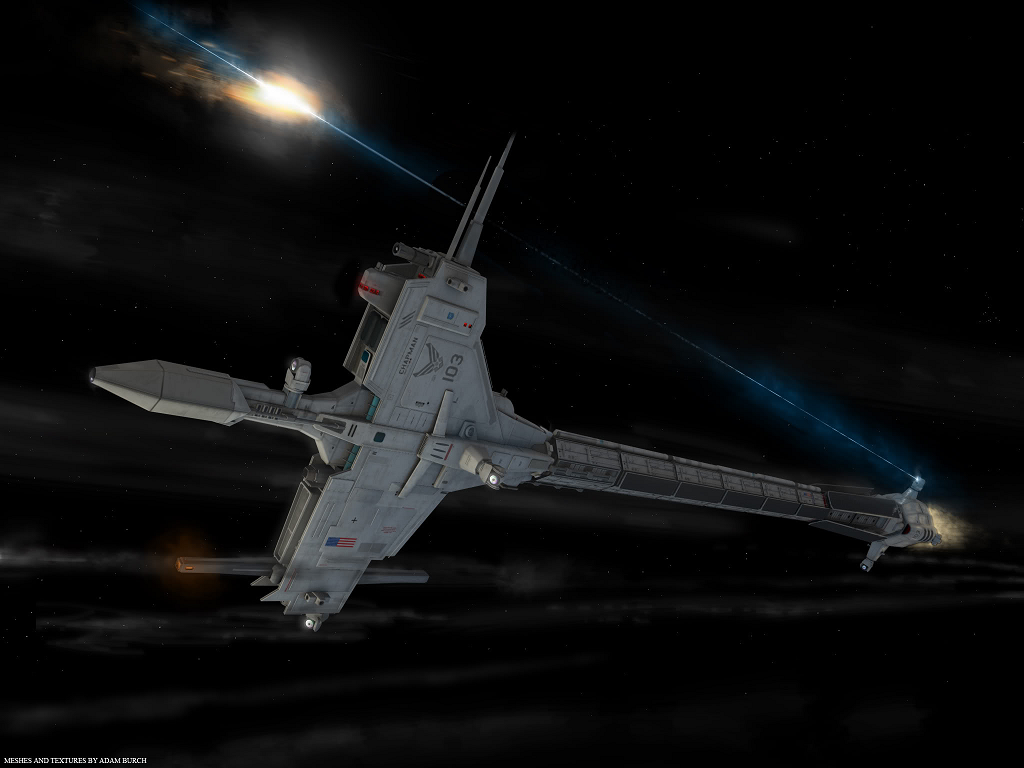Laser Frigate
At: 30
Df: 20
Mv: 25 days multiplied by (square root of distance in Astronomical Units), vacuum only
Cost: 16e+8r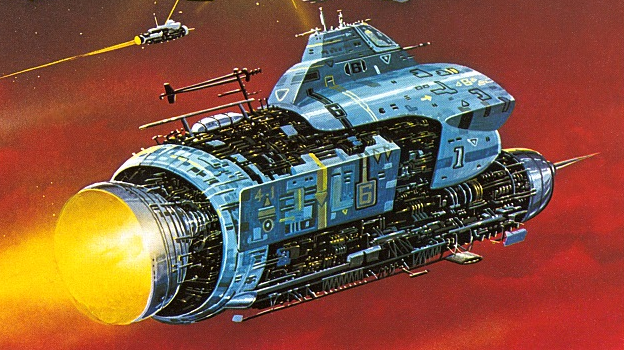Gunship
At: 35
Df: 25
Mv: 25 days multiplied by (square root of distance in Astronomical Units), vacuum only
Cost: 16e+16r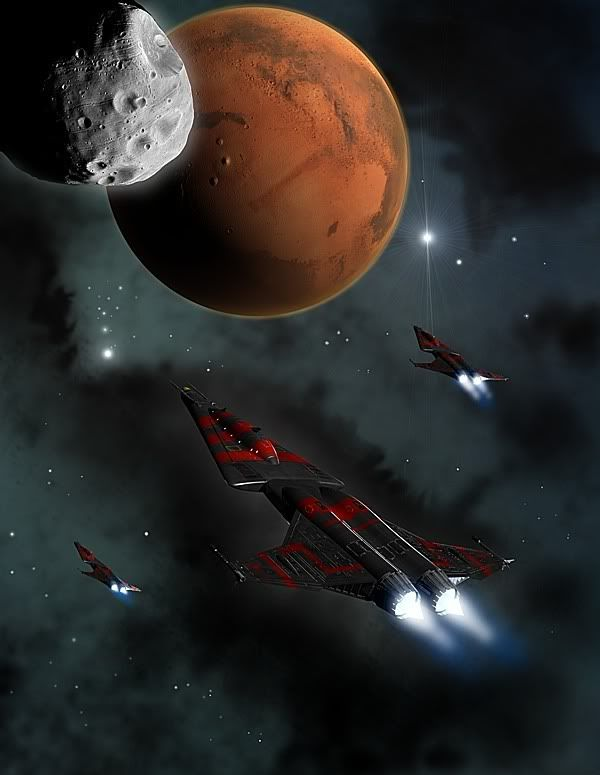Cruiser
At: 40
Df: 30
Mv: 25 days multiplied by (square root of distance in Astronomical Units), vacuum only
Cost: 20e+12r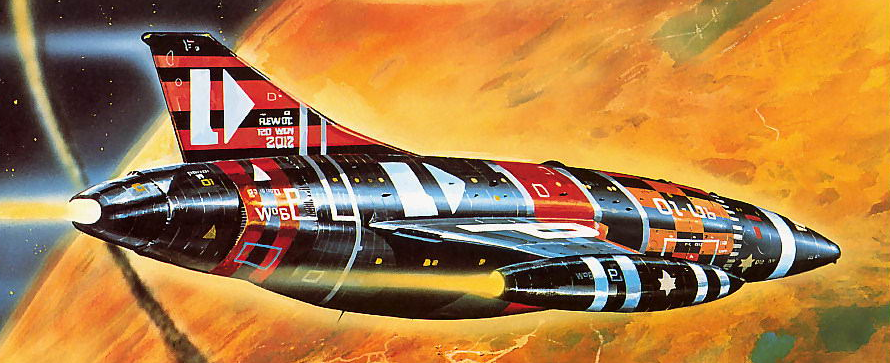Assault Ship
At: 40 (+10 against surface targets)
Df: 30
Mv: 25 days multiplied by (square root of distance in Astronomical Units), atmosphere capable
Cost: 24e+16r
Notes: Can carry up to 6 aircraft or Size Class A spacecraft

Size Class C:Battleship
At: 50
Df: 45
Mv: 25 days multiplied by (square root of distance in Astronomical Units), vacuum only
Cost: 28e+20r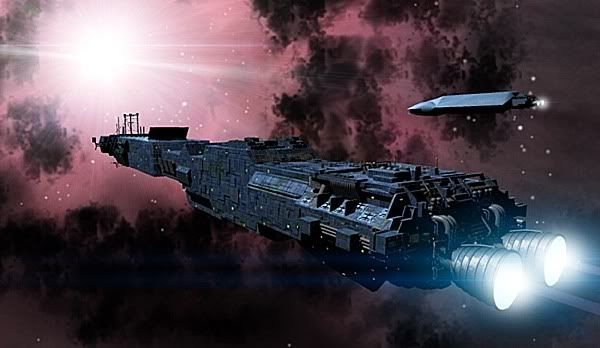Militarised Torchship/Picketstar
At: 25
Df: 100
Mv: 1G acceleration/deceleration, calculator here.
Cost: 15e+14r
Note: Has significant cargo space, and can carry up to 2 Size Class B vessels and 10 Size Class A craftFightstar
At: 35
Df: 110
Mv: 1G acceleration/deceleration, calculator here. Atmosphere capable.
Cost: 30e+30r
Note: Can carry up to 12 Espatiers or other infantry units

Size Class D:Battlestar
At: 50
Df: 125
Mv: 1G acceleration/deceleration, calculator here.
Cost: 60e+35r
Note: Can carry up to 4 Size Class B vessels and up to 25 Size Class A craftAt: 75
Df: 150
Mv: 1G acceleration/deceleration, calculator here.
Cost: 150e+50r
Note: Can carry up to 2 Size Class C vessels and up to 6 size class B vessels as well as up to 50 Size Class A craft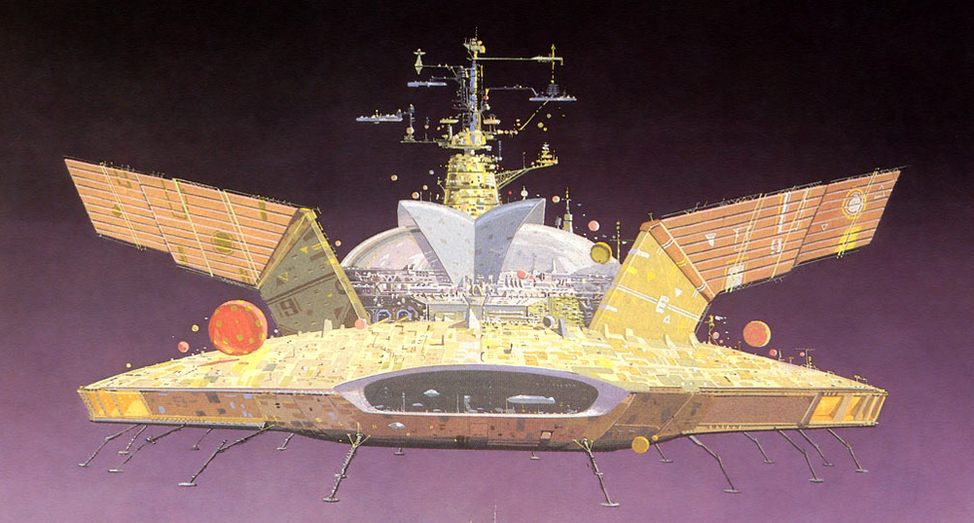Planetary Assault Vehicle
At: 100
Df: 50
Mv: 25 days multiplied by (square root of distance in Astronomical Units), atmosphere capable, surface lander
Cost: 120e+60r
Note: The PAV can carry up 2 landcrawler-sized units, 10 tank-sized units and 100 infantry units.Asteroid Fortress
At: 250
Df: 500
Mv: Orbit
Cost: 100e+50r
Note: Can also act as a base of operations for Space Force units.

NoXion
Posts: 556
Joined: Tue Jun 24, 2008 6:51 pm
Location: Nova Mundi

### Re: 22nd Century military units (Space Force)

Added the Asteroid Fortress.